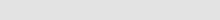Insilicase
deus ex computaGrams to addFinal volumeMaximum concentrationsStock solutionsOD to concOD to primer concProtein in DNA solnCentrifugation calculationsIsotope decayRadioactivity to molarityRadioactivity units conversionAny ideas?Contact me# Centrifugation calculations

This set of functions help to find the relative centrifugal force (g force) at a give rpm for a rotor and also the rpm needed for a certain g force. The calculations need the average radius of the rotor. This is normally stamped on the rotor, but can be calculated by adding the distance from the centre of the spindle to the furthest part of the centrifuge tube to the distance between the centre point and the nearest point of the tube and then dividing by 2.

#### RPM to RCF

Revolutions per minute (RPM)
Average radius of the rotor (cm)

#### RCF to RPM

Relative centrifugal force (g)
Average radius of the rotor (cm)

Back